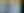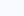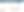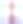Roel van Bommel

# The Box Plot: A gem, hidden in plain sight

First question: Wouldn’t it be nice to be able to know where churn is the most likely in your organization? Or to know which customers fall below a statistical minimum so you can specifically target them? Or even to go as far as to improve your packaging and placement of products in your warehouse based on outliers?
We bet you do!
Second question: Have you seen these kind of graphs in your dashboards lately?
We bet you don’t, or not often!In our experience, this lack of usage is mostly due to the fact that they need a bit of explanation before you know what to do with them.
This blog, written by Data Science experts, gives this explanation.
You’ll discover what a Box Plot is, how it works, and how you can use it within your dashboards.

## What is a Box Plot?

A Box Plot gives a good indication of how values in the data are spread out.
They provide a standardized way of displaying the distribution of data based on a five number summary (“minimum”, first quartile (Q1), median, third quartile (Q3), and “maximum”).• Median Value (Q2/50th Percentile)
Half the scores are greater than or equal to this value, and half the scores are smaller.
This value falls halfway between the total set of values and is also known as Q2 or 50th percentile. Also, this means that if the dataset has an even number of values, the average of the two middle values is used.

• First quartile (Q1/25th Percentile)
The median between the lowest value and the median value.

• Third quartile (Q3/75th Percentile)
The median between the highest value and the median value.

• Maximum
The outcome of the formula: Third quartile + (1.5*IQR).

• Minimum
The outcome of the formula: First quartile – (1.5*IQR).

• Interquartile range (IQR)
The IQR, middle 50% of the values, is calculated by determining the range between the first and third quartile.

• Outliers
Shown as the green circles in the visual above.

## Let’s simplify

The image below is a comparison of a Box Plot of a nearly normal distribution and the probability density function (pdf) for a normal distribution:Looking at a statistical distribution is more commonplace than looking at a Box Plot. In other words, it might help you understand a Box Plot.
The normal distribution is commonly associated with “the 68-95-99.7” rule:

• 68% of the data is within 1 standard deviation (σ) of the mean (μ)

• 95% of the data is within 2 standard deviations (σ) of the mean (μ)

• 99.7% of the data is within 3 standard deviations (σ) of the mean (μ).

This is where the term Six-Sigma comes from, which originated in the aviation safety industry.

For a Box Plot it’s sort alike on the calculations as most of the dashboarding tools take the probability density function as standard for their visualization. So:

• 50% of all Values are within the Interquartile range (IQR). That’s on 0,6745 of a standard deviation (σ) of the mean/median (μ).

• 24,65% of all Values are within the Minimum and first quartile (Q1).

• Also 24,65% of all Values are within the third quartile (Q3) and the Maximum.

• This leaves 0,7% of all values as outliers.

When you have a nearly normal distribution 7 out of 1000 values will be marked as outliers.

## How can a Box Plot assist the business?

All right, but what can it give me as a business? Let’s take these insights on a box plot and plot it on business processes. Like for example:

• Within HR you can plot departments per Area on In- & outflow.
This way, you can take action on outlier outflow departments.
Sidenote: please normalize your data before you use the Box Plot them; otherwise you end up with only the big departments being you outliers, it’s the percentage of in- & outflow that should be visualized.

• Within HR you can also plot employees per department on their age of working experience.
This way, you can see the working experience or age diversity over departments and detect outliers per department to prevent churn or to proactively adapt your workforce planning.

• Within Sales you can take a specific commodity SKU and plot weekly sales.
This way, you can check skewness and understand the buying pattern of your customers, which can develop into optimal pallet size & position in the warehouse of this SKU.

• Within Procurement you can plot buyers on “% On Contract Spend”.
This way, you can take action on outlier buyers that fall below the minimum and train them to upscale their process and contract knowledge.

• Within Sales Orders you can plot Carriers’ on Estimated Time of Arrival to determine when a Carrier is reliable enough on their expected delivery date within the PO. This to reliably update the confirmation date of the Sales Order for the customer when products are out of stock but in purchase.

There are many more examples of this, but the above situations are common to us.
Do you know that the Box plot is general available in SAP Analytics Cloud? We have used them in several of above given examples at our customers.

## Summary

This blog gave you a lot of information, so let’s summarize for a bit. A Box Plot:

• Visualizes the values of outliers.

• Helps identifying if data is symmetrical.

• Helps identifying how densely data is grouped.

• Helps identifying if your data is skewed.

• Displays this in a small but very clear visualization.

With the Box Plot, you can detect the outliers in your data and take immediate action. The Box Plot can give insight into the specific areas in your data that are unusually high or low.

## Let’s Connect!

We would like to simplify statistics for the business. In other words Team Business Science closes the gap between Business & Statistics. To apply statistics you first need to understand the Business. This all starts with the Problem Understanding & Data Understanding. You first need to understand before you can apply statistics upon it. Understand before you can be understood. And we like to help you with this.